# Python | Pandas dataframe.shift()

• Last Updated : 31 Aug, 2021

Python is a great language for doing data analysis, primarily because of the fantastic ecosystem of data-centric python packages. Pandas is one of those packages and makes importing and analyzing data much easier.
Pandas dataframe.shift() function Shift index by desired number of periods with an optional time freq. This function takes a scalar parameter called the period, which represents the number of shifts to be made over the desired axis. This function is very helpful when dealing with time-series data.

Syntax:DataFrame.shift(periods=1, freq=None, axis=0)
Parameters :
periods : Number of periods to move, can be positive or negative
freq : DateOffset, timedelta, or time rule string, optional Increment to use from the tseries module or time rule (e.g. ‘EOM’). See Notes
axis : {0 or ‘index’, 1 or ‘columns’}
Return : shifted : DataFrame

Example #1: Use shift() function to shift the index axis by 2 periods in a time-series data

## Python3

 `# importing pandas as pd``import` `pandas as pd`` ` `# Creating row index values for our data frame``# We have taken time frequency to be of 12 hours interval``# We are generating five index value using "period = 5" parameter`` ` `ind ``=` `pd.date_range(``'01 / 01 / 2000'``, periods ``=` `5``, freq ``=``'12H'``)`` ` `# Creating a dataframe with 4 columns``# using "ind" as the index for our dataframe``df ``=` `pd.DataFrame({``"A"``:[``1``, ``2``, ``3``, ``4``, ``5``],``                   ``"B"``:[``10``, ``20``, ``30``, ``40``, ``50``],``                   ``"C"``:[``11``, ``22``, ``33``, ``44``, ``55``],``                   ``"D"``:[``12``, ``24``, ``51``, ``36``, ``2``]},``                    ``index ``=` `ind)` `# Print the dataframe``df`

Output: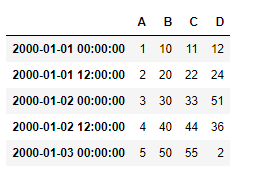Let’s use the dataframe.shift() function to shift the index axis by 2 periods in the positive direction

## Python3

 `# shift index axis by two periods in positive direction``# axis = 0 is set by default``df.shift(``2``, axis ``=` `0``)`

Output: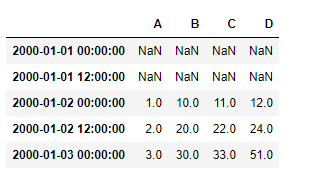Let’s shift the index axis in the negative direction by some periods

## Python3

 `# shift index axis by two periods in negative direction``# axis = 0 is set by default``df.shift(``-``2``, axis ``=` `0``)`

Output :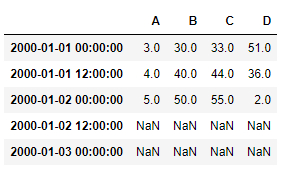Example #2: Use shift() function to shift the column axis by 2 periods in a time-series data

## Python3

 `# importing pandas as pd``import` `pandas as pd`` ` `# Creating row index values for our data frame``# We have taken time frequency to be of 12 hours interval``# We are generating five index value using "period = 5" parameter`` ` `ind ``=` `pd.date_range(``'01 / 01 / 2000'``, periods ``=` `5``, freq ``=``'12H'``)`` ` `# Creating a dataframe with 4 columns``# using "ind" as the index for our dataframe``df ``=` `pd.DataFrame({``"A"``:[``1``, ``2``, ``3``, ``4``, ``5``],``                   ``"B"``:[``10``, ``20``, ``30``, ``40``, ``50``],``                   ``"C"``:[``11``, ``22``, ``33``, ``44``, ``55``],``                   ``"D"``:[``12``, ``24``, ``51``, ``36``, ``2``]},``                    ``index ``=` `ind)` `# Print the dataframe``df`Let’s use the dataframe.shift() function to shift the column axis by 2 periods in a positive direction

## Python3

 `# shift column axis by two periods in positive direction``df.shift(``2``, axis ``=` `1``)`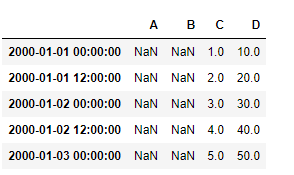Let’s shift the column axis in the negative direction by some periods

## Python3

 `# shift column axis by two periods in negative direction``df.shift(``-``2``, axis ``=` `1``)`

Output :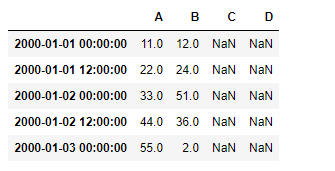My Personal Notes arrow_drop_up